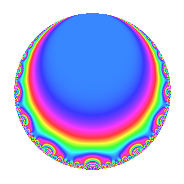Properties

 Label 189.2.a.eLevel 189 Weight 2 Character orbit 189.a Self dual Yes Analytic conductor 1.509 Analytic rank 0 Dimension 2 CM No Inner twists 2

Related objects

Newspace parameters

 Level: $$N$$ = $$189 = 3^{3} \cdot 7$$ Weight: $$k$$ = $$2$$ Character orbit: $$[\chi]$$ = 189.a (trivial)

Newform invariants

 Self dual: Yes Analytic conductor: $$1.5091725982$$ Analytic rank: $$0$$ Dimension: $$2$$ Coefficient field: $$\Q(\sqrt{3})$$ Coefficient ring: $$\Z[a_1, a_2]$$ Coefficient ring index: $$1$$ Fricke sign: $$-1$$ Sato-Tate group: $\mathrm{SU}(2)$

$q$-expansion

Coefficients of the $$q$$-expansion are expressed in terms of $$\beta = \sqrt{3}$$. We also show the integral $$q$$-expansion of the trace form.

 $$f(q)$$ $$=$$ $$q$$ $$+ \beta q^{2}$$ $$+ q^{4}$$ $$+ \beta q^{5}$$ $$+ q^{7}$$ $$-\beta q^{8}$$ $$+O(q^{10})$$ $$q$$ $$+ \beta q^{2}$$ $$+ q^{4}$$ $$+ \beta q^{5}$$ $$+ q^{7}$$ $$-\beta q^{8}$$ $$+ 3 q^{10}$$ $$-\beta q^{11}$$ $$+ 2 q^{13}$$ $$+ \beta q^{14}$$ $$-5 q^{16}$$ $$-4 \beta q^{17}$$ $$+ 5 q^{19}$$ $$+ \beta q^{20}$$ $$-3 q^{22}$$ $$+ \beta q^{23}$$ $$-2 q^{25}$$ $$+ 2 \beta q^{26}$$ $$+ q^{28}$$ $$-6 \beta q^{29}$$ $$+ 5 q^{31}$$ $$-3 \beta q^{32}$$ $$-12 q^{34}$$ $$+ \beta q^{35}$$ $$-7 q^{37}$$ $$+ 5 \beta q^{38}$$ $$-3 q^{40}$$ $$+ 3 \beta q^{41}$$ $$-4 q^{43}$$ $$-\beta q^{44}$$ $$+ 3 q^{46}$$ $$+ 4 \beta q^{47}$$ $$+ q^{49}$$ $$-2 \beta q^{50}$$ $$+ 2 q^{52}$$ $$+ 8 \beta q^{53}$$ $$-3 q^{55}$$ $$-\beta q^{56}$$ $$-18 q^{58}$$ $$-4 \beta q^{59}$$ $$+ 8 q^{61}$$ $$+ 5 \beta q^{62}$$ $$+ q^{64}$$ $$+ 2 \beta q^{65}$$ $$+ 14 q^{67}$$ $$-4 \beta q^{68}$$ $$+ 3 q^{70}$$ $$+ 3 \beta q^{71}$$ $$-4 q^{73}$$ $$-7 \beta q^{74}$$ $$+ 5 q^{76}$$ $$-\beta q^{77}$$ $$+ 8 q^{79}$$ $$-5 \beta q^{80}$$ $$+ 9 q^{82}$$ $$-6 \beta q^{83}$$ $$-12 q^{85}$$ $$-4 \beta q^{86}$$ $$+ 3 q^{88}$$ $$-5 \beta q^{89}$$ $$+ 2 q^{91}$$ $$+ \beta q^{92}$$ $$+ 12 q^{94}$$ $$+ 5 \beta q^{95}$$ $$-4 q^{97}$$ $$+ \beta q^{98}$$ $$+O(q^{100})$$ $$\operatorname{Tr}(f)(q)$$ $$=$$ $$2q$$ $$\mathstrut +\mathstrut 2q^{4}$$ $$\mathstrut +\mathstrut 2q^{7}$$ $$\mathstrut +\mathstrut O(q^{10})$$ $$2q$$ $$\mathstrut +\mathstrut 2q^{4}$$ $$\mathstrut +\mathstrut 2q^{7}$$ $$\mathstrut +\mathstrut 6q^{10}$$ $$\mathstrut +\mathstrut 4q^{13}$$ $$\mathstrut -\mathstrut 10q^{16}$$ $$\mathstrut +\mathstrut 10q^{19}$$ $$\mathstrut -\mathstrut 6q^{22}$$ $$\mathstrut -\mathstrut 4q^{25}$$ $$\mathstrut +\mathstrut 2q^{28}$$ $$\mathstrut +\mathstrut 10q^{31}$$ $$\mathstrut -\mathstrut 24q^{34}$$ $$\mathstrut -\mathstrut 14q^{37}$$ $$\mathstrut -\mathstrut 6q^{40}$$ $$\mathstrut -\mathstrut 8q^{43}$$ $$\mathstrut +\mathstrut 6q^{46}$$ $$\mathstrut +\mathstrut 2q^{49}$$ $$\mathstrut +\mathstrut 4q^{52}$$ $$\mathstrut -\mathstrut 6q^{55}$$ $$\mathstrut -\mathstrut 36q^{58}$$ $$\mathstrut +\mathstrut 16q^{61}$$ $$\mathstrut +\mathstrut 2q^{64}$$ $$\mathstrut +\mathstrut 28q^{67}$$ $$\mathstrut +\mathstrut 6q^{70}$$ $$\mathstrut -\mathstrut 8q^{73}$$ $$\mathstrut +\mathstrut 10q^{76}$$ $$\mathstrut +\mathstrut 16q^{79}$$ $$\mathstrut +\mathstrut 18q^{82}$$ $$\mathstrut -\mathstrut 24q^{85}$$ $$\mathstrut +\mathstrut 6q^{88}$$ $$\mathstrut +\mathstrut 4q^{91}$$ $$\mathstrut +\mathstrut 24q^{94}$$ $$\mathstrut -\mathstrut 8q^{97}$$ $$\mathstrut +\mathstrut O(q^{100})$$

Embeddings

For each embedding $$\iota_m$$ of the coefficient field, the values $$\iota_m(a_n)$$ are shown below.

For more information on an embedded modular form you can click on its label.

Label $$\iota_m(\nu)$$ $$a_{2}$$ $$a_{3}$$ $$a_{4}$$ $$a_{5}$$ $$a_{6}$$ $$a_{7}$$ $$a_{8}$$ $$a_{9}$$ $$a_{10}$$
1.1
 −1.73205 1.73205
−1.73205 0 1.00000 −1.73205 0 1.00000 1.73205 0 3.00000
1.2 1.73205 0 1.00000 1.73205 0 1.00000 −1.73205 0 3.00000
 $$n$$: e.g. 2-40 or 990-1000 Significant digits: Format: Complex embeddings Normalized embeddings Satake parameters Satake angles

Inner twists

Char. orbit Parity Mult. Self Twist Proved
1.a Even 1 trivial yes
3.b Odd 1 yes

Atkin-Lehner signs

$$p$$ Sign
$$3$$ $$1$$
$$7$$ $$-1$$

Hecke kernels

This newform can be constructed as the intersection of the kernels of the following linear operators acting on $$S_{2}^{\mathrm{new}}(\Gamma_0(189))$$:

 $$T_{2}^{2}$$ $$\mathstrut -\mathstrut 3$$ $$T_{5}^{2}$$ $$\mathstrut -\mathstrut 3$$